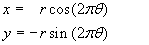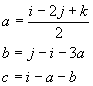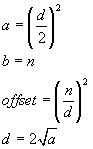NumberSpiral.com

CONTENTS9.   Formulas

To convert from polar coordinates to Windows screen coordinates (in which y increases from top to bottom, unlike conventional graphs):Note: theta is in rotations; x and y must be scaled and translated before using them for screen displayTo plot the spiral, including both the thin gray coiled line and the integers on it: :Note: theta is in rotationsTo plot offset curves:Note: theta is in rotationsTo derive coefficients a, b, c of a quadratic formula from three successive integers i, j, k in a quadratic sequence:For more information about these formulas including their derivation, see Method of Common Differences.To convert between a composite offset curve of angle n/d (measured in rotations) and its related quadratic function ax2 + bx:To factor an integer on a composite offset curve:To find factors on a product curve, where D is the difference between factors and n is an integer that varies from zero to infinity:Each integer on the product curve is equal to f1 f2.Here is another function for the same purpose, except this one calculates the factors as a function of any real number x on the spiral:1  2  3  4  5  6  7  8  9  10  Next